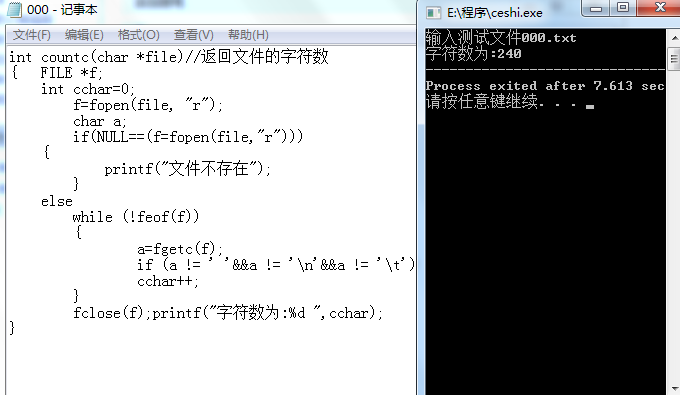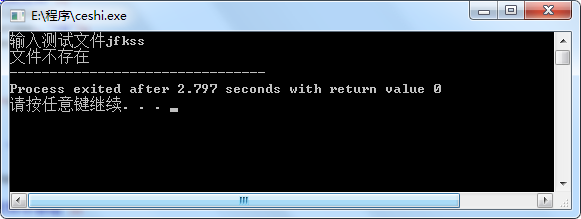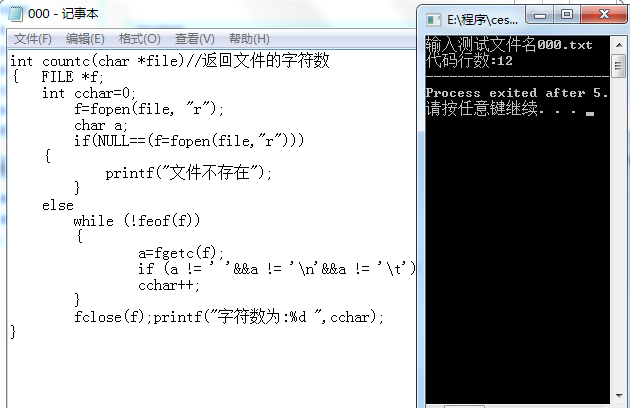# 一.互审代码情况

本次项目我与合作者采用的是c语言做的。在代码互审前，我首先上网查询许多有关c语言代码的规范以及风格，并对此展开了初略的了解与学习，并以此总结了一些自己喜欢且适合自己的C语言代码风格规范。

在代码互审时，我发现我和我的合作者两人都存在着许多问题。最大的问题就是我们两人的代码都写得比较随意，代码没有一个规范的结构，给人一种很乱的感觉，可读性较差。其次就是里面的变量名取的比较随意，没有实际的意义。这些都是我们代码存在的一些不好的东西。所以我们两通过查阅资料，了解一些正规的代码规范，并加我们的代码进行以改正。

# 二.代码实现

以下给出了扩展功能的实现情况：

空行数的统计实现

int count_blankline(char *file) //返回文件的空行数
{   FILE *f;
int b_num = 0;
int ch_num = 0;
char ch;
f = fopen(file, "r");
if(NULL==(f=fopen(file,"r")))
{
printf("file is NULL");
}
else
while (!feof(f))
{
ch= fgetc(f);
if (ch=='\n'){
if (ch_num<= 1)
b_num++;
ch_num = 0;
}
else if (ch!=' '&&ch!='\t'&&ch!='}')
ch_num++;
else if(ch=='}')b_num++;
}
fclose(f);printf("空行数:%d  ",b_num);
}

注释行数统计的实现：

int count_noteline(char *file) //返回文件的注释行数
{   FILE *f;
int ch_num = 0;int note_num=0;
char ch;
f=fopen(file, "r");
if(NULL==(f=fopen(file,"r")))
{
printf("文件不存在");
}
else
while (!feof(f))
{
ch= fgetc(f);
if(ch=='\n'){if(ch_num==2) note_num++; ch_num=0;}
else if(ch=='/') ch_num++;
else if(ch_num==1){if(ch=='/') ch_num++;}
}
fclose(f);
printf("注释行:%d  ",note_num);
} 

文件的遍历实现：

int searchfile(void) //寻找文件夹中的txt文件
{
struct _finddata_t filefind;
long handle;
int t=0;

if( (handle=_findfirst( "d:\\wordcount\\*txt", &filefind)) == -1L )
{
printf( "没找到txt文件\n");
}
else
do{
t++;
printf("找到文件:%s\n", filefind.name);
}while (_findnext(handle,&filefind)==0);

_findclose(handle);

printf("txt文件数量：%d\n",t);
return 0;
}

代码行数统计实现：

int count_codeline(char *file)//返回文件的代码行数
{   int ch_num = 0;
int code_num=0;
FILE *f;
int tag=0; int flag=0;
char a;
f = fopen(file, "r");
if(NULL==(f=fopen(file,"r")))
{printf("文件不存在");}
else
while (!feof(f))
{
a=fgetc(f);

if(flag==2) {
flag=0;tag++;}
else{

if(a=='\n'&&ch_num>1)
{code_num++;
ch_num=0;    }
else if(a != ' '&&a != '\n'&&a != '\t'&&a!='/') {
ch_num++;}

else if(a=='/'){ flag++;}
}

}

fclose(f); printf("代码行数:%d  ",code_num-tag);
}

运行结果如下：

# 三.测试

该项目的代码由许多函数组成，且函数的结构都差不多，只是统计的东西不一样。每一个函数在调用时都是传入的一个文件地址然后进行统计，在这里我对某些函数进行了单独测试。

字符数的统计函数测试代码

#include<stdio.h>
int countc(char *file);
int main ()
{
FILE *fpt;
char filename;
printf("输入测试文件");
scanf("%s",&filename) ;
fpt=fopen(filename, "rb");
countc(filename);
return 0;
}#include<stdio.h>
int count_codeline(char *file);
int main ()
{
FILE *fpt;
char filename;
printf("输入测试文件名");
scanf("%s",&filename) ;
fpt=fopen(filename, "rb");
count_codeline(filename);
return 0;
}# 四.项目总结

我觉得该项目对于我们来是一次非常好的锻炼，因为这个项目虽然不是一个很大的项目，但却是一个比较完整的项目。所谓麻雀虽小，五脏俱全，通过该项目，让我们完整具体的去体验了一次项目开发与测试过程。当然在项目的实现过程中，还是会遇到一些困难。但现在这个时代是一个信息的时代，许多困难都可以通过互联网去解决，实在不行还可以询问请教同学和老师。该项目在实现要考虑的东西还是很多的。例如你要统计一个东西，你必须要弄清楚这种东西形成的条件，这样你才能在混乱的稳当当中提取出你要统计的东西。

posted on 2018-10-21 19:50  BZMN  阅读(141)  评论(0编辑  收藏  举报